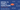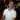# How to use Excel's Find Function

October 25, 2017

When working with text, sometimes you need to look for certain characters in order to parse out pieces of a string. With Excel’s `FIND()` function, you can get the position of the character (or characters) that you’re looking for.

## Definition and Syntax

The `FIND()` function searches for a specific string within some text and returns the position of where it found the string.

Note that the starting index of the text being searched on is 1.

``FIND(find_text, within_text [, start_num])``
Parameter Definition
`find_text` This is the text you will be searching for.
`within_text` This is the text that you will be searching in.
`start_num` Optional. This is the starting position within the text where you want to begin searching the `find_text`.## Other Notes

• `FIND()` is case sensitive. This means that if you look for “sally” (lower case) it will return an error (#VALUE!).
• If `find_text` is not found within the string, #VALUE! is returned.
• If `find_text` is blank or missing, like `=FIND(,A1)`, it will return `1` (the first position of the string).
• If `start_num` is outside the index range of the string, #VALUE! is returned. For example if we have the string “Hello” where the indexes of the string are 12345 (1=H, 2=e, 3=l, etc.) and we put a number in start_num that is not within those numbers, #VALUE! is returned.
• You can’t use wildcards with the `FIND()` function. For that, you would want to use the `SEARCH()` function.

## Examples

For the following examples we’ll use this text:

``Sally sells sea shells by the sea shore. The shells Sally sells are surely from the sea.``

### Find the First Word in a Sentence

``````=FIND(" ",A1)
=6``````

The first space found in the text is at position `6`. We can then combine this info with the LEFT() function to get the first word of the sentence.

``````=LEFT(A1,FIND(" ",A1)-1)
=Sally``````

Note that the `-1` is in the formula to remove the trailing space, so instead of returning `"Sally "` it returns `"Sally"`.

### Find the Second Word in a Sentence

To get the second word in a sentence, we’ll have to use the MID() function in combination with two FIND() functions: one to get the first space and another to get the second space.

Before we begin, remember that the syntax for MID() is:

``=MID(text, start_num, num_chars)``

The idea here is to get the start_num where the second word begins. Then we need the amount of characters the second word is to put into the num_chars parameter.

``````Text: Sally sells ...
Index:123456789abc... (where a=10, b=11, and c=12)
Goal: Start at index 7 (start_num) and get 5 characters (num_chars)

=MID(A1, FIND(" ",A1)+1, FIND(" ",A1,FIND(" ",A1)+1)-FIND(" ",A1)-1)
--  --------------  ------------------------------------------
text   start_num                   num_chars

Note: Each underlined section represents what is being evaluated

1) =MID(A1, FIND(" ",A1)+1, FIND(" ",A1, FIND(" ",A1)+1)  -FIND(" ",A1)-1)
------------
2) =MID(A1, 6+1,            FIND(" ",A1, FIND(" ",A1)+1)  -FIND(" ",A1)-1)
---
3) =MID(A1, 7,              FIND(" ",A1, FIND(" ",A1)+1)) -FIND(" ",A1)-1)
-                            ---------------
4) =MID(A1, 7,              FIND(" ",A1, 7)               -FIND(" ",A1)-1)
------------ --
5) =MID(A1, 7,              12                            -FIND(" ",A1)-1)
--                             ------------
6) =MID(A1, 7,              12                            -6-1)
--                            ----
7) =MID(A1,7,5)
-----------
8)=sells``````

#### Getting the Second Word - Breakdown

I know, I know, this is pretty confusing. Let’s break this down into the steps to provide a more clear explanation.

1. The whole formula. We also show the parts that we are looking to get for the `MID()` function.
2. We start with getting the start_num for `MID()`. The first `FIND()` function is looking for the first space.
3. The first space evaluates to `6`.
4. The `start_num` evaluates to `7`, which is the “s” in “sells”. Now let’s start evaluating the `num_chars` parameter for the `MID()` function. We’ll start by evaluating getting the first space `+1`.
5. As before, it evaluates to `7`. Now we are looking for the second space’s position. Note that we are looking for a space after the first space was found at position `6` (we have to `+1` position `6` to ignore the same space for the `FIND` function).
6. We get `12` for the position of the second space.
7. Now we want to get the position of the first space again because we’re trying to get the number of characters for the second word (which is `5` characters).
8. Again, getting the first space is at position `6`.
9. Evaluating `12-6-1 = 5`.
10. We finally get the second word “sells” without any beginning or trailing spaces.

As you can imagine, getting the 3rd or 4th word can get a little hairy. If you need to get the first N words from a string, check out this post.

Wow, you read the whole article! You know, people who make it this far are true learners. And clearly, you value learning. Would you like to learn more about Excel? Please consider supporting me by buying me a coffee (it takes a lot of coffee to write these articles!).Written by Joseph who loves teaching about Excel.